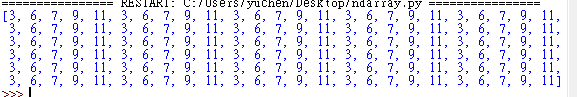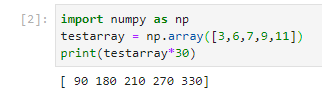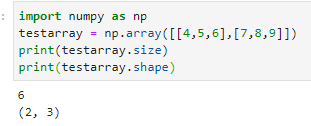#DAY 4
1

## Numpy

Numpy 是 Python 的一個模組，主要用於資料處理，

1.ndarray 如果我們今天有一個矩陣想要他數字的30倍

``````testarray = [3,6,7,9,11]
print(testarray*30)

````````````import numpy as np #插入模組
testarray = np.array([3,6,7,9,11])
print(testarray*30)

``````(不是我不用idle 是找模組裝好麻煩，偷偷靠向jupyter )

NumPy 可以透過 .size 與 .shape來知道2d矩陣的規模

``````import numpy as np
testarray = np.array([[4,5,6],[7,8,9]])
print(testarray.size)#看大小
print(testarray.shape)#看外觀
``````### 1 則留言

1